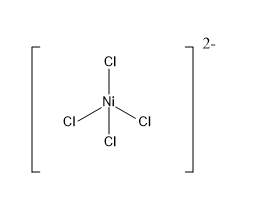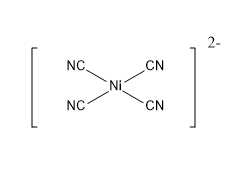Courses
Courses for Kids
Free study material
Offline Centres
MoreLast updated date: 30th Nov 2023
Total views: 279k
Views today: 4.79k

# What is the geometry of complexes having hybridization $s{p^3}$ and $ds{p^2}$ . Give an example of each.Verified
279k+ views
Hint: Hybridization is the mixing of atomic orbitals with the same energy to form a new degenerate orbital. The orbital thus formed is called the hybrid orbital. Hybrid orbitals possess different geometry and energy than that of the individual atomic orbitals. $sp\,,s{p^2}\,,s{p^3}\,,s{p^3}{d^2},{d^2}sp$ etc. are examples of few hybridised orbitals.

Atomic orbitals of equal energy mix to form a hybrid orbital. Both half-filled and completely filled orbitals can take part in hybridisation. The number hybrid orbitals will be equal to the number of atomic orbitals that took part in mixing.
$s{p^3}$ hybridization: It is formed by the mixing of one s orbital and three p orbitals of the same shell of an atom that are equal in energy. Mixing of these orbitals give $4$ equivalent hybrid orbitals with $s{p^3}$ hybridization. An $s{p^3}$ hybridised orbital contains $25\%$ s character and $75\%$ p character. The geometry of complexes possessing $s{p^3}$ hybridization will be tetrahedral. The four atoms bound to the central atom will be directed towards the four corners of the tetrahedron. The bond angle will be ${109^ \circ }28'$ .
Example: ${[Ni{(Cl)_4}]^{2 - }}$ .$ds{p^2}$ hybridization: Mixing of ${d_{{x^2} - {y^2}}}$ orbital, one s orbital and two p orbitals of equivalent energies gives four equivalent $ds{p^2}$ $( + 2)$ hybridised orbitals. An $ds{p^2}$ hybridized orbital contains $25\%$ d character, $25\%$ s character and $50\%$ p character. The geometry of a $ds{p^2}$ hybridised complex is square planar. The bond angle between the ligand-central atom-ligand will be ${90^ \circ }$ .
Example : ${[Ni{(CN)_4}]^{2 - }}$Note:
Though the central atom and its oxidation state $( + 2)$ are the same in both the complexes, the difference in the geometry of the complex is due to the nature of ligands. Strong ligands will form $ds{p^2}$ complexes, while weak ligands will form $s{p^3}$ hybridised complexes.
It should be noted that molecular orbitals and hybrid orbitals are not the same. Molecular orbitals are formed by the interaction of different atomic orbitals while hybrid orbitals are formed by the mixing of atomic orbitals of the same atom.